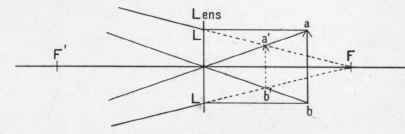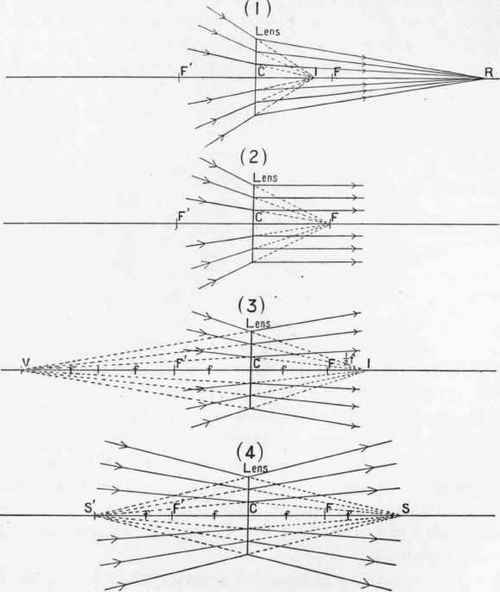From Figs. 30 and 31 we see that as the object approaches the lens from infinity the size of the virtual image increases and approaches the lens. If the object be brought up to the plane coinciding with the focal point f, or even nearer to the lens, the same order of things continues, until the object is in contact with the lens, or coincides with the centre of the lens; here image and object both coincide, and we have the position for unit magnification for a negative lens.

To impress this, as the case differs from a positive lens, we will illustrate the case when the object is nearer to the lens than its focal point on the same side.

Taking our two rays whose paths are known: - a l, parallel to the axis, emerges as though it originated at f, and a c passes through the centre of the lens ; they cut one another at a', forming the virtual image of a. Similarly b' is the virtual image of b, and a' b' that of a b.

Our conclusion from the above observation is: that a real object placed anywhere between the lens and infinity forms a virtual image somewhere between the centre of the lens and its focal point on the same side. And conversely : Convergent rays incident upon a negative lens, proceeding to a focus situated anywhere between the centre of the lens and its focal point on the other side, will form a real image somewhere between the centre of the lens and infinity on that side.

If, however, convergent rays meeting a negative lens are proceeding to a focus anywhere beyond the focal point on the other side no real image can be formed by the negative lens.

Fig.32.If we examine the convergent pencils (2), Fig. 33, proceeding towards the focal point f, we see that they emerge parallel to the axis, to form an image at infinity. It is easy to see that if they converged to a point beyond F they would no longer emerge parallel, but become divergent, and thus could not form a real image.

As a matter of interest let us now illustrate the facts arrived at in a comparative manner.

In (1) the rays converge towards a point anywhere between the lens and the focal point f ; a real image is formed at r.

In (2) the rays converge towards the focal point f and emerge parallel.

In (3) the rays converge towards a point 1, beyond the focal point f ; they diverge, as from its conjugate, a virtual image at v, and no real image is formed.

Fig.33.In (4), similarly, the rays converge towards the symmetric point s (beyond the focal point), diverging from the lens at the same inclination as the incident rays, and as though they originated in the virtual symmetric point s'.

If we reverse (3) we see the conditions for convergent rays proceeding to a point beyond the symmetric point, and in general confirm our conclusion that no real image can be formed by a negative lens when the rays converge to a point beyond its focal point on that side of the lens.

We give in conclusion a practical method of determining the focal length of a negative lens with accuracy:-

1. Focus accurately the image of some well-defined object with any ordinary positive lens, and measure the size of the image.

2. Place the negative lens, the focal length of which we wish to determine, a short distance within the convergent beam from the positive lens. Then focus the image accurately formed by the combined lenses and measure its size. Note:

(a) The distance of the screen from any fixed point on the mounting of the negative lens; call this d.

(b) The magnification that has occurred to the image formed by the positive lens alone; call this m.

3. Now move the negative lens a little nearer to the positive lens (n.b. - Keep the positive lens in a fixed position), and focus accurately a second time upon the screen, and as before note:

(c) The distance of the screen from the same fixed point on the mounting of the negative lens; call this d'.

(d) The magnification of the image now formed by the positive lens alone; call this m'.

The focal length of negative lens = D' - D/M'-M

## Notes

The four "cardinal points" of a negative lens, as in the case of a positive lens, are the two principal points, and the two focal points. Here again the principal points are a certain distance apart, dependent upon the thickness and form of the lens, and possess the property that light proceeding from any direction towards one of them passes out from the lens as though it had passed through the other. The relation f1 n1 = f2 n2 in Fig. 34 always holds good, so that we may trace the course of rays through a thick lens by plotting the two principal planes passing through the two principal points in the axis, and the two focal points on either side.

Fig.34.Fig.35.A ray a p1 passing from a parallel to the axis (Fig. 35) meets the focal plane p1 n1 in p: and emerges from p2 as though it originated at f1 : similarly the ray a n2 meeting the principal point n1 emerges from n2 in a direction parallel to a n1i.e., in the direction a n2 ; the point a, where

These three photographs are all untouched, the normal-sized eyes are those of children. Taken with the same Telephoto combination as Plates II., III., and V., at a distance of 4 feet m all three cases. The magnified adult eye was obtained by using a greater extension of camera. Exposure, small eyes, 3 seconds in an ordinary room; large eye 25 seconds in a badly lighted studio. (By the Author.) n2 a meets p2 f1 determines the position of the virtual image a of the object a.

In the same manner we can find the image of any other point in the object a b, and thus determine the position of the entire virtual image a b.

The relation between the size of the object a b and its image a b is found by our interpretation of the law of conjugate foci: xy = f2

As image and object are here both on the same side of the lens x= n1b + f, and y = b f ; so that when x = n + 1 times f, y=1 /n+1 f.

To take a numerical example : suppose f = 2 inches, and the object is 10 inches from it; here n = 5 times, so that x = (5 + 1) 2 and y = (1/5+1) 2; f2 =xy

= 12 x 1/3 f2=4 f = 2.

Conversely, in examining a real image formed by a negative lens of 2 inches focal length used in conjunction with any positive system, we find this real image is 10 inches distant from the negative lens, and know that it has produced a magnification (very nearly) of 10/2 + 1 or 6 times.

In dealing with thick lenses, the measurement should of course be taken from the principal point of emergence, so that a slight error may arise. The position of the principal points in negative lenses differs with their form and thickness.

In the double concave lens a (Fig. 36) both are contained within the glass ; in a plano-concave b one is always on the axis where the concave surface cuts it; and in the negative meniscus, one may fall within the lens or both may be outside, as shown in c and d.

In d we note that the ray a b proceeding to n1, and which passes through the centre of the lens (i.e., towards c), emerges from it as c d parallel to a b and passes through the other principal point n2.

Fig. 36.In the construction of negative lens-systems used in practice, the principal points do not generally lie outside the lenses, so that only very small or negligible errors come in when determining "magnification" by the above process.